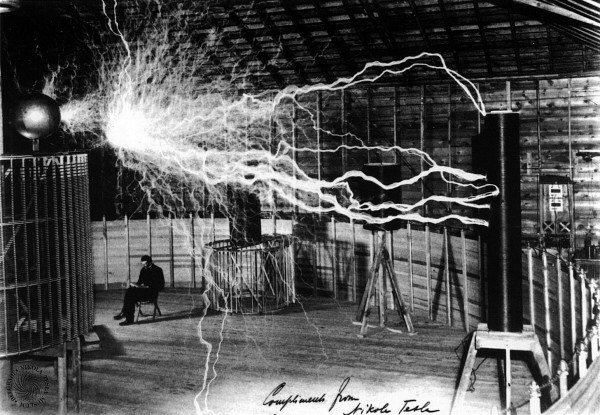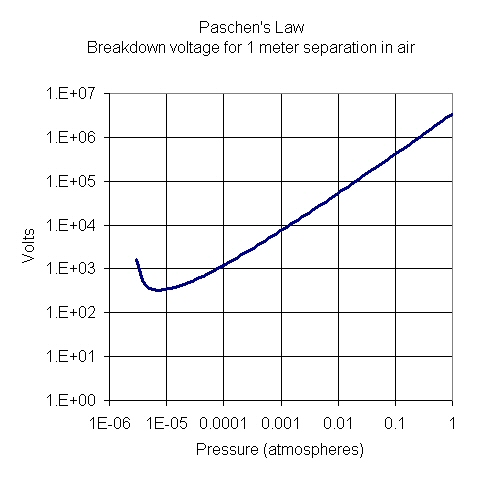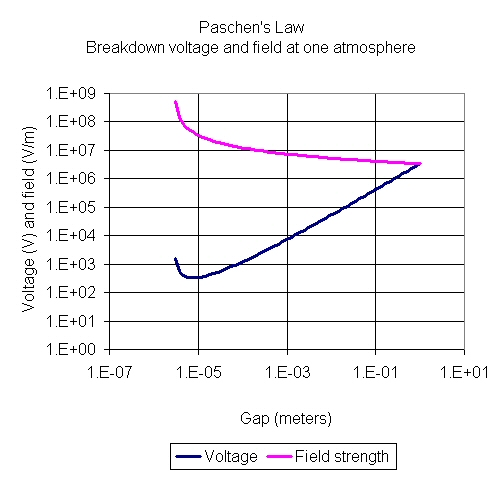Power Handling

Click here to go to our page on switch FET power handlingThe voltage of Nicola Tesla's man-made lightning can be calculated from altitude and gap

When we talk about power handling, we mean power levels beyond which damage occurs (the magic blue smoke is released), usually well beyond the dynamic range of a system. Typical power handling issues are receiver protection during transmit for a system that the transmitter and receiver are duplexed to the same antenna. But any lab course should consider this subject, because exceeding power handling limitations of expensive test equipment can cost a lot of money!

Two limitations generally exist.

• Average power kills hardware through heating effects
• Peak power kills hardware through breakdown effects (like arcing)

By the way, this seemingly simple subject of power handling quite often requires very complex analyses, you can make a career out of it!

Average power

In this case we need to consider the combined heat due to average RF and DC power forms, and heat transfer to a heat sink. The better the heat sink, the more power you can apply.

The analysis of heat transport is a complex subject, and we are not going to get into it in any detail now...

Peak power

In this case we need to consider the combined maximum voltage due to RF and DC power forms, while voltage limits must be applied to peak waveforms.

Ionization breakdown in air occurs when a critical field strength is exceeded. Field strength is specified in volts/meter or volts per centimeter. In air, at 1 atmosphere, the critical field is often quoted at about 3,000,000 volts/meter, or 30,000 volts/cm, or about 76 volts per mil. Generally, this breakdown voltage is NOT a function of frequency, but it is a strong function of altitude and gap size.

One way to greatly improve power handling is to add a dielectric in place of air. Therefore, coax cables with PTFE fill have far greater power handling than air coax. The same same goes for stripline. For high-voltage applications that must work at high altitude (such as microwave tube power supplies for airborne radar) the entire part is often "potted" with a dielectric filler, this often makes repair impossible.

One thing to look out for is that when you mate connectors, even if they are filled with dielectric, the interface can have just a slight air gap. Guess where voltage breakdown is going to occur?

Paschen's Law

Paschen's Law dates back to the 19th century, named after Friedrich Paschen, and is often referenced when in voltage breakdown calculations. For this effort you will find Friedrich Paschen in the Microwave Hall of Fame!

The empirical equation that Paschen came up describes the relationship between voltage breakdown, distance and gas pressure:

Vb=(apd)/(ln(pd)+b)

The constants a and b take on different values for different gases, For dry air, a=43.6*106 and b=12.8. Vb is the breakdown voltage in Volts, p is the pressure, d is the gap distance. Given these constants, the units of p (pressure) are atmospheres and d (distance) are meters.An approximate number for breakdown electric field is 3 million volts per meter, or 30,000 volts per centimeter, for a gap of 7 mm at 1 atmosphere. Quoting an exact value for breakdown in air is not possible unless you consider gap size, pressure, and humidity.... even the geometry of the electrodes matters. Paschen's law applies to spherical electrodes.

Peak power handling in waveguide

Waveguide power handling is subject to both heat (average power) and voltage breakdown (peak power) limitations.

Voltage breakdown in rectangular waveguide at sea-level air pressure can be predicted by the following approximate equation:

Pbr=600,000*a*b*(Lambda/Lambdaguide) (watts) Equation 1

where a and b and in centimeters.

Lambdaguide is explained here. To take a step back further in equation land, Pbr may be written as a function of the breakdown field strength (although we don't have an exact reference for where this came from, except some PowerPoint found on the web by Roy...)

Pbr=6.63E-4*a*b*(Lambda/Lambdaguide)*(Ebr)^2 (watts) Equation 2

where Ebr is in volts/cm, a and b are in centimeters.

In this case you will notice that the Ebr value that corresponds to 600,000 value in Equation 1 is 30,000 volts/cm which represents a 7mm gap at one atmosphere.

If you plug in the dimensions for WR-90, the power handling is in excess of 1 megawatt at band center where lambda/lambdaguide is 0.75. Yikes!

For circular waveguide, the approximate power handling equation becomes:

Pbr=450,000d^2(Lambda/Lambdaguide) (watts)

where d is diameter in centimeters.

These two equations came from page 70 of Gershon Wheeler's excellent book, Introduction to Microwaves, circa 1963. Look for Wheeler's book on our book page! Note that his information on the critical field for breakdown probably dated to WWII and more accurate numbers are now available. If knowing the exact power handling of a particular waveguide is important to you, you need to dive into this topic a little more than we have. Be sure to consider altitude effects on Ebr!

Waveguide power handling can be increased by pressurizing, or using certain inert gases which can withstand higher much electric fields, such as freons.

Update September 2013: thanks to Roy for pointing out we did not provide the units for a, b and d in the above equations!

Multipaction

This topic is discussed on a separate page.

Semiconductor failure

Failure can occur in semiconductors, when breakdown occurs in the "channel" or "junction". Voltages must be kept below a critical threshold in semiconductor devices or failure results. The voltage is instantaneous voltage, the sum of DC and RF components.

Author : Unknown Editor